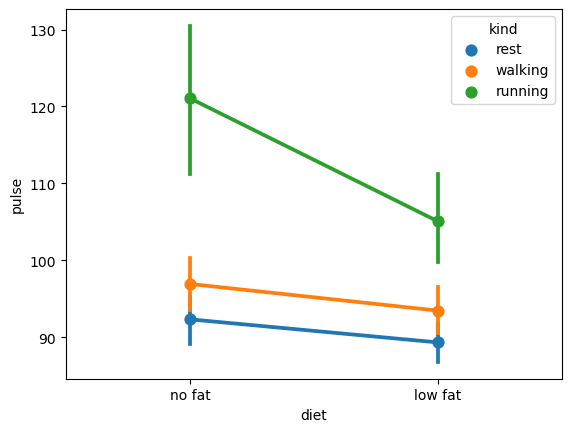# Seaborn Seaborn Pandas

In :
import numpy as np
import pandas as pd
from scipy import stats
import matplotlib.pyplot as plt
import seaborn as sns

In :
df_obj1 = pd.DataFrame({"x": np.random.randn(500), "y": np.random.randn(500)})
df_obj1

Out:
x y
0 -0.935656 -2.521335
1 0.644625 -0.847422
2 -1.269324 0.271522
3 -0.675344 0.788331
4 0.274915 1.834406
... ... ...
495 -0.469763 0.338594
496 0.573595 0.960775
497 1.032013 1.003825
498 -0.926715 -0.700403
499 -1.194451 -0.022291

500 rows × 2 columns

In :
df_obj2 = pd.DataFrame({"x": np.random.randn(500),
"y": np.random.randint(0, 100, 500)})
df_obj2

Out:
x y
0 -1.715442 61
1 -0.966911 9
2 0.931460 12
3 -0.523918 10
4 -1.270239 85
... ... ...
495 1.620159 29
496 -2.090487 82
497 2.321468 29
498 0.898830 17
499 -0.826900 1

500 rows × 2 columns

In :
help(sns.jointplot)

Help on function jointplot in module seaborn.axisgrid:

jointplot(data=None, *, x=None, y=None, hue=None, kind='scatter', height=6, ratio=5, space=0.2, dropna=False, xlim=None, ylim=None, color=None, palette=None, hue_order=None, hue_norm=None, marginal_ticks=False, joint_kws=None, marginal_kws=None, **kwargs)
Draw a plot of two variables with bivariate and univariate graphs.

This function provides a convenient interface to the :class:JointGrid
class, with several canned plot kinds. This is intended to be a fairly
lightweight wrapper; if you need more flexibility, you should use
:class:JointGrid directly.

Parameters
----------
data : :class:pandas.DataFrame, :class:numpy.ndarray, mapping, or sequence
Input data structure. Either a long-form collection of vectors that can be
assigned to named variables or a wide-form dataset that will be internally
reshaped.
x, y : vectors or keys in data
Variables that specify positions on the x and y axes.
hue : vector or key in data
Semantic variable that is mapped to determine the color of plot elements.
Semantic variable that is mapped to determine the color of plot elements.
kind : { "scatter" | "kde" | "hist" | "hex" | "reg" | "resid" }
Kind of plot to draw. See the examples for references to the underlying functions.
height : numeric
Size of the figure (it will be square).
ratio : numeric
Ratio of joint axes height to marginal axes height.
space : numeric
Space between the joint and marginal axes
dropna : bool
If True, remove observations that are missing from x and y.
{x, y}lim : pairs of numbers
Axis limits to set before plotting.
color : :mod:matplotlib color <matplotlib.colors>
Single color specification for when hue mapping is not used. Otherwise, the
plot will try to hook into the matplotlib property cycle.
palette : string, list, dict, or :class:matplotlib.colors.Colormap
Method for choosing the colors to use when mapping the hue semantic.
String values are passed to :func:color_palette. List or dict values
imply categorical mapping, while a colormap object implies numeric mapping.
hue_order : vector of strings
Specify the order of processing and plotting for categorical levels of the
hue semantic.
hue_norm : tuple or :class:matplotlib.colors.Normalize
Either a pair of values that set the normalization range in data units
or an object that will map from data units into a [0, 1] interval. Usage
implies numeric mapping.
marginal_ticks : bool
If False, suppress ticks on the count/density axis of the marginal plots.
{joint, marginal}_kws : dicts
Additional keyword arguments for the plot components.
kwargs
Additional keyword arguments are passed to the function used to
draw the plot on the joint Axes, superseding items in the
joint_kws dictionary.

Returns
-------
:class:JointGrid
An object managing multiple subplots that correspond to joint and marginal axes
for plotting a bivariate relationship or distribution.

--------
JointGrid : Set up a figure with joint and marginal views on bivariate data.
PairGrid : Set up a figure with joint and marginal views on multiple variables.
jointplot : Draw multiple bivariate plots with univariate marginal distributions.

Examples
--------

.. include:: ../docstrings/jointplot.rst


In :
sns.jointplot(x = "x", y = "y", data = df_obj2)

Out:
<seaborn.axisgrid.JointGrid at 0x248cd5f1460>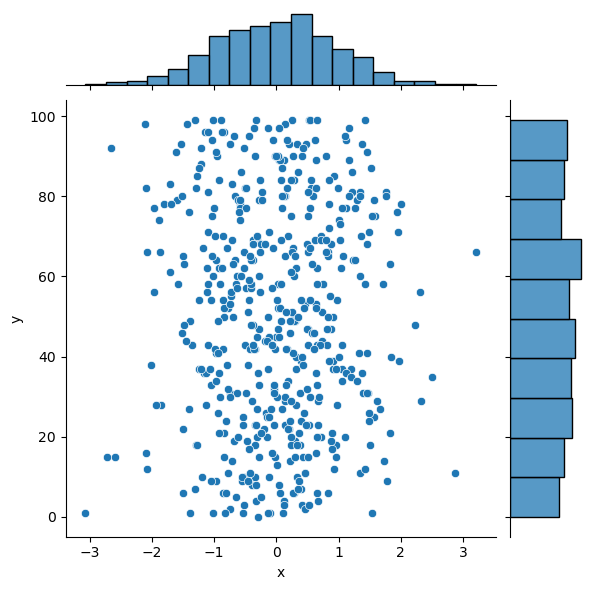In :
sns.jointplot(x = "x", y = "y", data = df_obj2, kind = "hex");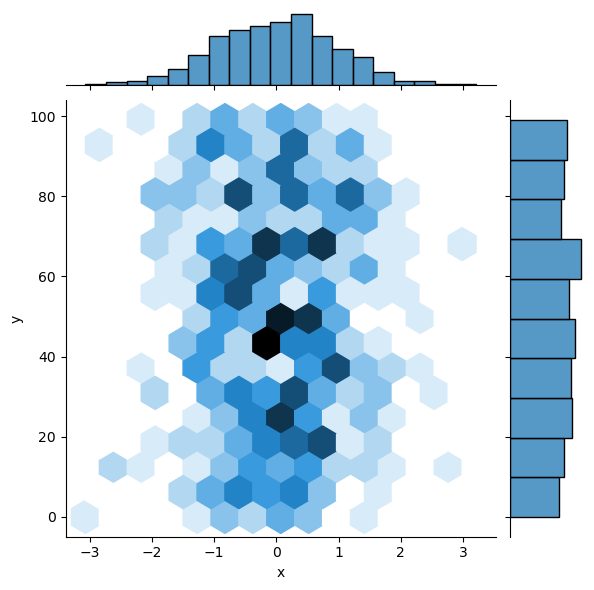In :
sns.jointplot(x = "x", y = "y", data = df_obj1, kind = "kde");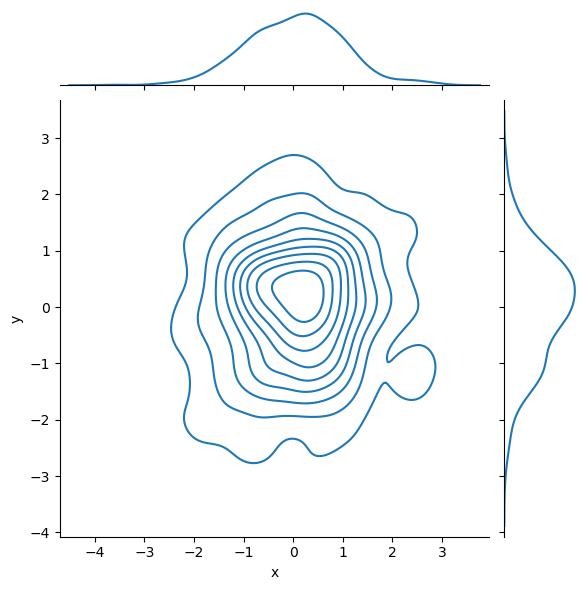In :
dataset = sns.load_dataset("tips")
sns.pairplot(dataset);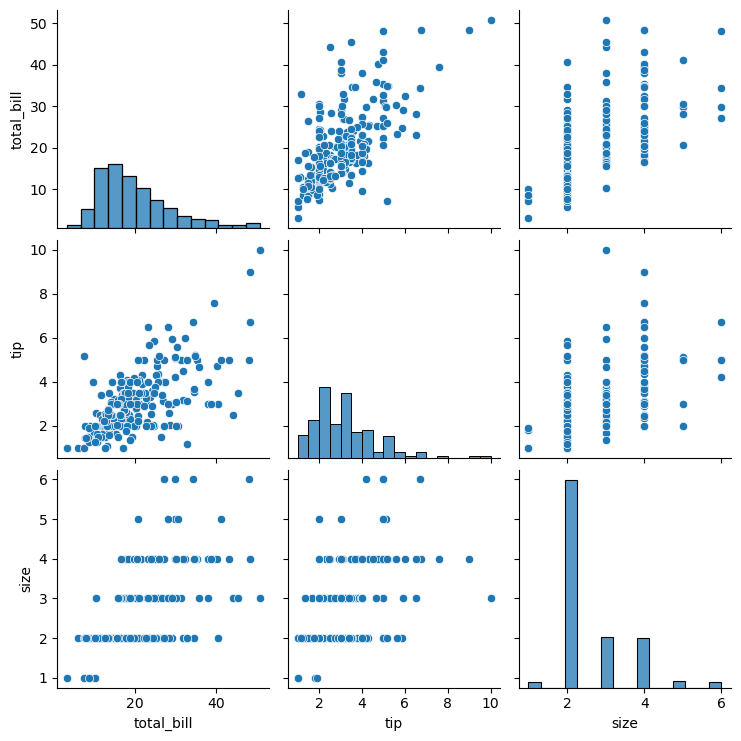In :
#titanic = sns.load_dataset('titanic')

In :
exercise = sns.load_dataset('exercise')
exercise

Out:
Unnamed: 0 id diet pulse time kind
0 0 1 low fat 85 1 min rest
1 1 1 low fat 85 15 min rest
2 2 1 low fat 88 30 min rest
3 3 2 low fat 90 1 min rest
4 4 2 low fat 92 15 min rest
... ... ... ... ... ... ...
85 85 29 no fat 135 15 min running
86 86 29 no fat 130 30 min running
87 87 30 no fat 99 1 min running
88 88 30 no fat 111 15 min running
89 89 30 no fat 150 30 min running

90 rows × 6 columns

In :
sns.stripplot(x="diet", y="pulse", data=exercise)

Out:
<AxesSubplot:xlabel='diet', ylabel='pulse'>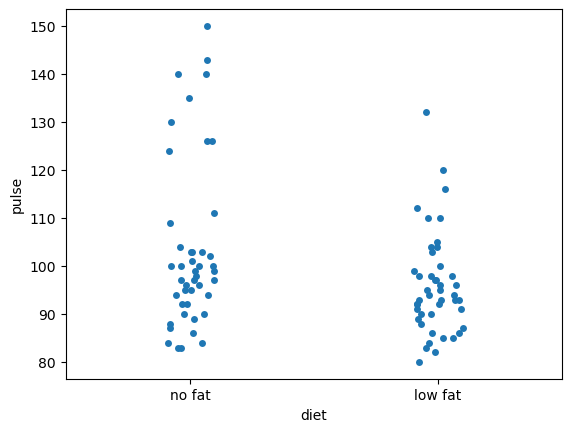In :
sns.swarmplot(x="diet", y="pulse", data=exercise, hue='kind')

Out:
<AxesSubplot:xlabel='diet', ylabel='pulse'>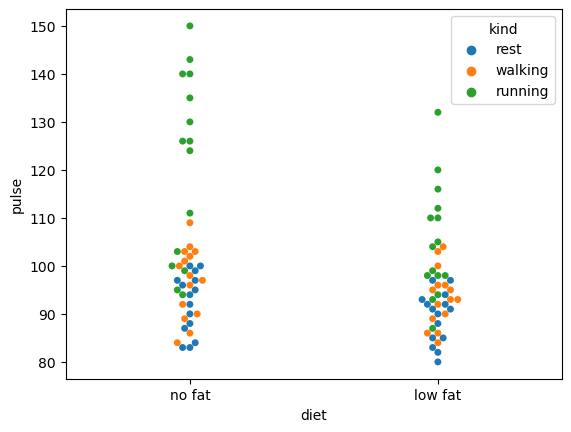In :
sns.boxplot(x="diet", y="pulse", data=exercise)

Out:
<AxesSubplot:xlabel='diet', ylabel='pulse'>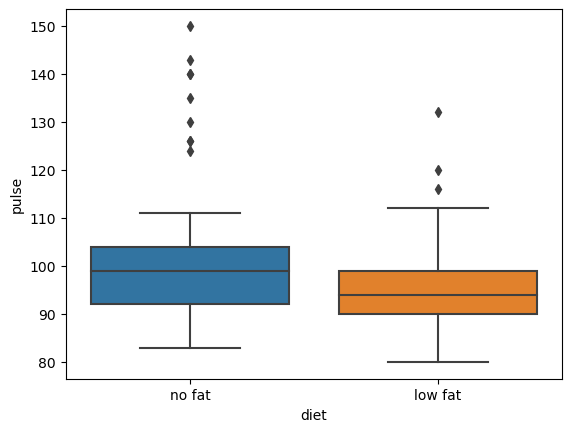In :
sns.boxplot(x="diet", y="pulse", data=exercise, hue='kind')

Out:
<AxesSubplot:xlabel='diet', ylabel='pulse'>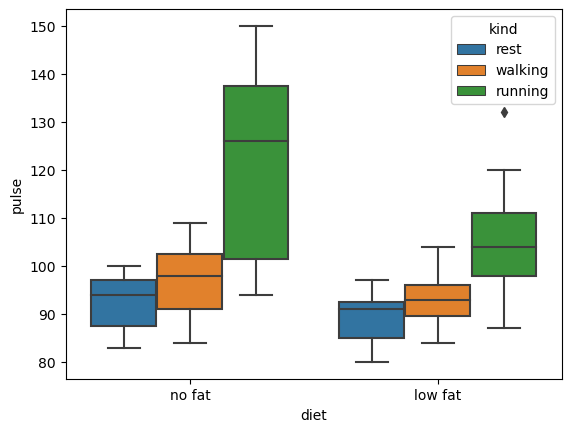In :
sns.violinplot(x="diet", y="pulse", data=exercise, hue='kind')

Out:
<AxesSubplot:xlabel='diet', ylabel='pulse'>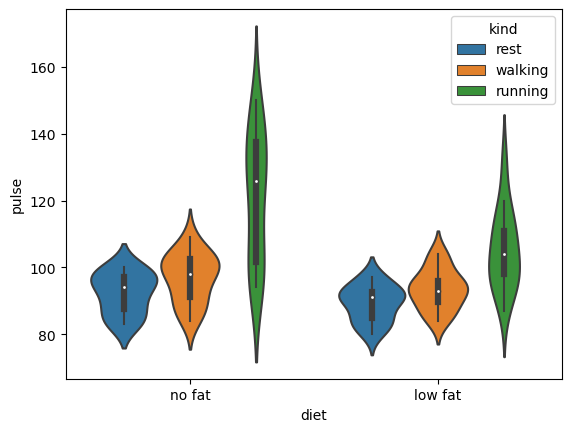In :
sns.barplot(x="diet", y="pulse", data=exercise, hue='kind')

Out:
<AxesSubplot:xlabel='diet', ylabel='pulse'>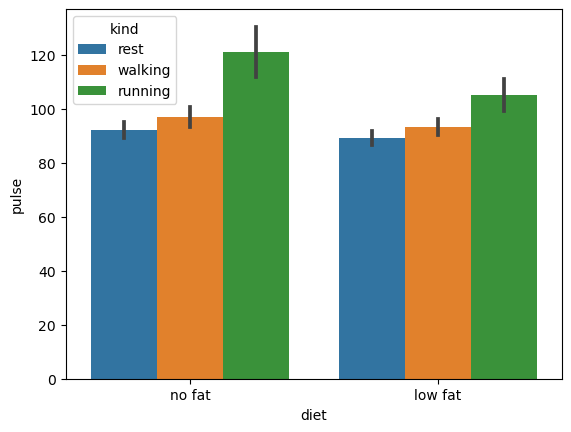In :
sns.pointplot(x="diet", y="pulse", data=exercise, hue='kind');